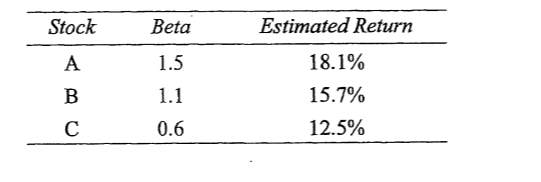Scan QR code or get instant email to install app

Question:

# An analyst gathered the following data about three stocks:If the risk-free rate is 8%, and the market risk premium is 7%, the analyst is least likely to recommend buying:A Stock A.
explanation

Stock A: kA = 8% + 1.5(7%) = 18.5%. Because the estimated return of 18.1% is less than the required return of 18.5%, Stock A is overvalued.
Stock B: kB - 8% + 1.1(7%) = 15.7%. Because the estimated return of 15.7% equals the required return of 15-7%, Stock B is properly valued.
Stock C: kc = 8% + 0.6(7%) = 12.2%. Because the estimated return of 12.5% is greater than the required return of 12.2%, Stock C is undervalued.